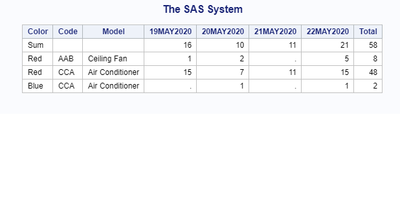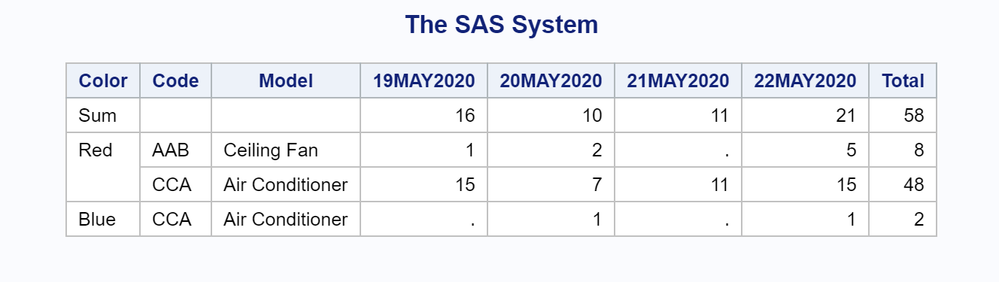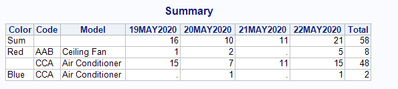## Proc Report make 2 rows align into 1 row and create a total column

Hello,

Hoping if someone can please help me and let me know how to make 2 rows align into 1 row and create a total column?

this is what I have :

 19-May-20 20-May-20 21-May-20 22-May-20 Color Code Model Total Total Total 16 10 11 21 Red AAB Ceiling Fan 1 2 . 5 Red CCA Air Conditioner 15 7 11 15 Blue CCA Air Conditioner . 1 . 1

proc report data=Summary_Vol  nowd;

column color Code Model  Day , Volume;

define color  / group 'color' ;

define Code / group 'Code';

define Model / group 'Volume';

define Day  / across '';

define Volume / analysis sum '';

COMPUTE segment;

IF color NE ' ' then hold=color ;

IF color EQ ' ' then color=hold ;

ENDCOMP;

rbreak before / summarize;

compute before;

if color = "" then color = 'Total';

if Code = "" then Code = 'Total';

if Model = "" then Model = 'Total';

ENDCOMP;

run;

This is what I want to get:

 Color Code Model 19-May-20 20-May-20 21-May-20 22-May-20 Total Total Total Total 16 10 11 21 58 Red AAB Ceiling Fan 1 2 . 5 8 Red CCA Air Conditioner 15 7 11 15 48 Blue CCA Air Conditioner . 1 . 1 2

1 ACCEPTED SOLUTION

Accepted Solutions

## Re: Proc Report make 2 rows align into 1 row and create a total column

You almost had it.  Here you go!

`proc report data=WORK.import nowd ;column color Code Model Volume, Day(Volume=tot);define color / group order=data;define Code / group ;define Model / group ;define Day / across ' ';define Volume / analysis sum '' ;define tot / analysis sum 'Total' f=6.0 ;COMPUTE color;IF not missing(color) then hold=color ;IF missing(color) then color=hold ;ENDCOMP;rbreak before / summarize;compute before;color='Sum';endcomp;run;`9 REPLIES 9

## Re: Proc Report make 2 rows align into 1 row and create a total column

Hi @sufiya

Could you please supply raw data?

best,

## Re: Proc Report make 2 rows align into 1 row and create a total column

Hi @ed_sas_member  & @ghosh  - attached the raw data- thank you both!

## Re: Proc Report make 2 rows align into 1 row and create a total column

Hi @ghosh  - uploaded csv version - thank you again!

## Re: Proc Report make 2 rows align into 1 row and create a total column

It's pretty simple, you have done most of the work. Can you provide the data so I could test it out?

## Re: Proc Report make 2 rows align into 1 row and create a total column

`proc report data=work.import nowd spanrows;column color Code  Model Volume, Day			  (Volume=tot);define color  / group order=data;define Code / group ;define Model / group ;define Day  / across ' ';define Volume / analysis sum '' ;define tot / analysis sum 'Total'  f=6.0 ;rbreak before / summarize;compute before; color='Sum'; endcomp;run;`## Re: Proc Report make 2 rows align into 1 row and create a total column

Hi @ghosh

Thank you  - it worked! I  have a question, I want to have the first column 'Color' variables repeat but they don't  - how can I update the query for this?

was adding to get column variables to repeat:

``````COMPUTE color;
IF color NE ' ' then hold=color ;
IF color EQ ' ' then color=hold ;
ENDCOMP;``````

full code:

``````proc report data=WORK.RawData nowd spanrows;
column color Code Model Volume, Day
(Volume=tot);
define color / group order=data;
define Code / group ;
define Model / group ;
define Day / across ' ';
define Volume / analysis sum '' ;

define tot / analysis sum 'Total' f=6.0 ;
COMPUTE color;
IF color NE ' ' then hold=color ;
IF color EQ ' ' then color=hold ;
ENDCOMP;

rbreak before / summarize;
compute before;
color='Sum';
endcomp;

run;

ODS HTML CLOSE;
quit;``````## Re: Proc Report make 2 rows align into 1 row and create a total column

You almost had it.  Here you go!

`proc report data=WORK.import nowd ;column color Code Model Volume, Day(Volume=tot);define color / group order=data;define Code / group ;define Model / group ;define Day / across ' ';define Volume / analysis sum '' ;define tot / analysis sum 'Total' f=6.0 ;COMPUTE color;IF not missing(color) then hold=color ;IF missing(color) then color=hold ;ENDCOMP;rbreak before / summarize;compute before;color='Sum';endcomp;run;`## Re: Proc Report make 2 rows align into 1 row and create a total column

thank you!
Discussion stats
• 9 replies
• 798 views
• 1 like
• 3 in conversation